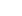Laboratoire Angevin de Recherche en Ingénierie des Systèmes

Séparés par des virgules

## TEXTURE MODELING WITH SCATTERING TRANSFORMS

Sixin ZHANG

Abstract :

We study how to model the texture as a stationary and ergodic random process. The texture is assumed to be homogenous in two-dimensional space, i.e. stationary under translation, and is ergodic in sense that the statistics do not differ considerably from one single realization to another. These statistical properties fit well the classical microcanonical modeling framework, resulting in a maximum entropy process satisfying a pre-defined set of statistics. The main challenge is to define this set of statistics so as to capture the non-Gaussianarity of the underlying random process, in particular when the texture has long-range dependency and complex geometry such as vorticity solutions two-dimensional Navier-Stokes equation. One common way to evaluate the model is to synthesis texture of similar visual quality and variability. We use the scattering transform to capture the geometric correlations in the space beyond second order statistics. The basic idea is to model progressively the Gaussian and non-Gaussian part of the random process through a cascade of convolutional and non-linear transforms. When the random process is a Gaussian process, we use the l2 moments of the 1st order scattering coefficients to approximate its covariance structure. We evaluate the Gaussian model by standard metrics in power spectrum estimation. The use of the Gabor wavelets in 1st order scattering also extracts the non-Gaussian statistics. The non-linear modulus operator is then applied to introduce the correlations between across scales and angles. We then build a second order model with PCA to capture these non-Gaussian correlations by the 2nd order scattering coefficients. We will present results on the synthesis texture of Turbulence and Brodatz texture dataset.

Sixin ZHANG is a postdoc in the Data Processing and Classification team lead by Stéphane MALLAT at Dépt Informatique, ENS Paris – CNRS. Sixin ZHANG completed his PhD in 2016, on “Distributed stochastic optimization for deep learning”, at the Courant Institute of Mathematical Sciences, NYU, USA, under the supervision of Yann LECUN. Sixin ZHANG obtained a Master degree in Mathematics and Machine Learning, at Paris VI and ENS Cachan (2008-2010).